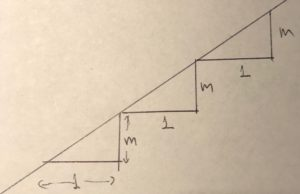By William McCallum

In my last post I wrote about the following standard, and mentioned that I could write a whole blog post about the first comma.

8.F.A.3. Interpret the equation $y = mx + b$ as defining a linear function, whose graph is a straight line; give examples of functions that are not linear. For example, the function $A = s^2$ giving the area of a square as a function of its side length is not linear because its graph contains the points $(1,1)$, $(2,4)$ and $(3,9)$, which are not on a straight line.

The comma indicates that the clause “whose graph is a straight line” is nonessential for identifying the noun phrase “linear function.” It turns the clause into an extra piece of information: “and by the way, did you know that the graph of a linear function is a straight line?” This fact is often presented as obvious; after all, if you draw the graph or produce it using a graphing utility, it certainly looks like a straight line.

When I’ve asked prospective teachers why this is so, I’ve gotten answers that look something like this:

We know that a linear function has a constant rate of change, $m$. If you go across by 1 on the graph you always go up by $m$, like this:So the graph is like a staircase. It always goes up in steps of the same size, so it’s a straight line.

This is fine as far as it goes. It identifies the defining property of a linear function—that it has a constant rate of change—and relates that property to a geometric feature of the graph. But it’s a “Here, Look!” proof. In the end it is showing that something is true rather than showing why it is true. Which is to say that it’s not a proof.

Still, the move to a geometric property of linear functions is a move in the right direction, because it focuses our minds on the essential concept. We all know that any two points lie on a line, but three points might not. What is it about three points on the graph of a linear function that implies they must lie on a straight line?Line from $A$ to $B$ to $C$ is dotted because we don’t know it’s a line yet

Because a linear function has a constant rate of change, the slope between any two of the three points $A$, $B$, and $C$ is the same. So $|BP|/|AP| = |CQ|/|AQ|$, which means there is a scale factor $k =|AQ|/|AP| = |CQ|/|BP|$ so that a dilation with center $A$ and scale factor $k$ takes $P$ to $Q$, and take the vertical line segment $BP$ to a vertical line segment based at $Q$ with the same length as $CQ$. Which means it must take $B$ to $C$.

But (drumroll) this means that there is a dilation with center $A$ that takes $B$ to $C$. Dilations always take points on a ray from the center to other points on the same ray. So $A$, $B$, and $C$ lie on the same line.

I don’t really expect students to get all of this, at least not right away. I’d be happy if they understood that there is a geometric fact at play here; that seeing is not always believing.##### William McCallum
Founder and CEO at | Website

Bill McCallum, founder of Illustrative Mathematics, is a University Distinguished Professor of Mathematics at the University of Arizona. He has worked in both mathematics research, in the area of number theory and arithmetical algebraic geometry, and mathematics education, writing textbooks and advising researchers and policy makers. He is a founding member of the Harvard Calculus Consortium and lead author of its college algebra and multivariable calculus texts. In 2009–2010 he was one of the lead writers for the Common Core State Standards in Mathematics. He holds a Ph. D. in Mathematics from Harvard University and a B.Sc. from the University of New South Wales.

## 2 thoughts on “Why is the graph of a linear function a straight line?”

1.hpicciotto says: